### dragontalesHover over the thumbnail for a full-size version.

Author NICNAC14 author:nicnac14 n-art rated 2008-11-06 2008-11-06 4 by 7 people. \$dragontales#NICNAC14#none#00000000000000000000000000000000000000000000000000000000000000000000000000000000000000000000000000000000000000000000000000000000000000000000000000000000000000000000000000000000000000000000000000000000000000000000000000000000000000000000000000000000000000000000000000000000000000000000000000000000000000000000000000000000000000000000000000000000000000000000000000000000000000000000000000000000000000000000000000000000000000000000000000000000000000000000000000000000000000000000000000000000000000000000000000000000000000000000000000000000000000000000000000000000000000000000000000000000000000000000000000000000000000000000000000000000000000000000000000000000000000000000000000000000000000000000000000000000000000000|5^492,480!5^252,216!10^504,504!10^507,501!10^516,488!10^526,485!10^527,476!10^521,467!10^513,462!10^506,460!10^483,451!10^475,451!10^461,453!10^471,453!10^452,456!10^497,503!10^489,502!10^474,500!10^478,500!10^462,499!10^453,499!10^333,480!10^323,476!10^321,467!10^322,460!10^337,455!10^348,457!10^322,456!10^332,456!10^346,451!10^329,434!10^329,434!10^315,406!10^298,365!10^287,321!10^445,458!10^437,462!10^438,455!10^447,451!10^455,445!10^463,434!10^475,417!10^486,397!10^480,374!10^458,360!10^430,368!10^402,388!10^368,360!10^385,341!10^410,321!10^440,307!10^379,331!10^290,275!10^475,432!10^496,415!10^520,396!10^531,370!10^532,338!10^528,308!10^520,283!10^524,248!10^541,246!10^559,245!10^442,154!10^432,163!10^429,180!10^430,197!10^435,212!10^442,226!10^449,244!10^449,260!10^469,287!10^457,283!10^472,288!10^468,288!10^464,285!10^463,284!10^458,284!10^456,283!10^454,282!10^454,282!10^451,280!10^446,279!10^446,279!10^445,278!10^440,275!10^440,275!10^435,270!10^433,269!10^432,269!10^431,268!10^427,266!10^425,264!10^424,264!10^418,263!10^415,262!10^413,262!10^407,261!10^404,262!10^404,262!10^398,262!10^392,262!10^388,262!10^384,263!10^382,263!10^382,264!10^376,264!10^370,267!10^362,270!10^358,273!10^354,275!10^352,276!10^351,278!10^351,279!10^351,279!10^353,275!10^361,271!10^363,269!10^368,267!10^372,265!10^375,264!10^379,263!10^346,280!10^346,280!10^342,289!10^338,292!10^334,301!10^333,305!10^333,305!10^337,297!10^344,285!10^350,276!10^329,305!10^326,314!10^326,314!10^326,316!10^325,322!10^325,330!10^324,331!10^324,328!10^328,322!10^327,328!10^332,325!10^333,321!10^335,317!10^338,314!10^342,312!10^346,312!10^351,312!10^352,315!10^353,320!10^354,324!10^355,328!10^355,331!10^355,329!10^355,323!10^360,319!10^363,315!10^367,311!10^372,309!10^375,309!10^381,309!10^385,314!10^386,315!10^387,316!10^387,316!10^388,316!10^390,311!10^391,307!10^394,301!10^399,299!10^400,298!10^403,297!10^408,297!10^411,297!10^415,297!10^417,300!10^420,292!10^430,288!10^436,288!10^441,288!10^442,287!10^445,289!10^452,292!10^455,299!10^423,289!10^423,289!10^429,286!10^433,286!10^443,288!10^448,297!10^572,228!10^508,245!10^490,238!10^476,229!10^466,216!10^468,199!10^479,182!10^515,199!10^534,204!10^553,210!10^573,214!10^508,183!10^590,208!10^606,196!10^615,181!10^560,157!10^546,149!10^527,140!10^518,141!10^514,126!10^503,111!10^482,503!10^474,499!10^468,498!10^464,498!10^459,498!10^456,498!10^460,498!10^490,502!10^490,502!10^494,503!10^499,503!10^501,503!10^506,503!10^508,501!10^508,499!10^508,495!10^508,494!10^509,491!10^510,489!10^518,484!10^518,484!10^523,484!10^527,476!10^526,471!10^522,469!10^519,466!10^519,466!10^514,465!10^507,464!10^500,462!10^495,457!10^488,455!10^482,454!10^481,453!10^476,453!10^464,453!10^460,453!10^452,456!10^446,459!10^436,460!10^446,499!10^445,499!10^439,498!10^433,496!10^428,495!10^422,494!10^418,494!10^415,494!10^415,494!10^406,493!10^403,493!10^399,492!10^394,491!10^391,491!10^388,491!10^388,491!10^385,491!10^373,488!10^367,486!10^363,485!10^357,485!10^350,484!10^344,483!10^339,483!10^338,483!10^345,483!10^357,485!10^368,491!10^374,492!10^380,492!10^383,492!10^325,477!10^325,477!10^318,474!10^318,470!10^317,463!10^321,458!10^330,454!10^341,455!10^350,459!10^350,459!10^355,459!10^358,461!10^362,461!10^354,457!10^348,454!10^344,447!10^340,445!10^338,443!10^333,438!10^331,433!10^328,433!10^327,432!10^325,427!10^318,419!10^317,415!10^316,414!10^316,414!10^325,427!10^326,428!10^322,418!10^318,408!10^317,408!10^316,407!10^311,401!10^307,394!10^306,388!10^304,386!10^300,382!10^294,371!10^296,376!10^304,384!10^305,391!10^311,395!10^315,403!10^296,367!10^295,366!10^295,363!10^292,357!10^292,357!10^290,353!10^285,344!10^285,340!10^284,335!10^284,330!10^283,327!10^283,322!10^284,317!10^284,317!10^282,306!10^282,298!10^281,293!10^281,288!10^282,284!10^284,280!10^288,271!10^289,269!10^289,283!10^281,308!10^281,308!10^281,315!10^281,322!10^281,331!10^282,341!10^284,344!10^288,351!10^288,356!10^292,264!10^292,260!10^292,254!10^294,248!10^296,243!10^299,239!10^300,239!10^300,236!10^300,229!10^301,227!10^303,223!10^306,218!10^309,213!10^310,209!10^311,206!10^365,261!10^365,260!10^364,256!10^362,252!10^358,247!10^358,242!10^358,239!10^358,235!10^358,232!10^358,231!10^359,229!10^359,222!10^359,218!10^361,211!10^361,209!10^361,205!10^361,202!10^361,201!10^362,199!10^362,191!10^361,184!10^361,178!10^360,175!10^356,171!10^355,170!10^354,168!10^352,163!10^348,158!10^346,156!10^341,149!10^338,146!10^333,144!10^328,143!10^328,143!10^324,143!10^317,141!10^313,141!10^309,142!10^308,142!10^303,143!10^300,144!10^297,145!10^308,201!10^309,200!10^310,196!10^310,192!10^305,189!10^303,190!10^297,191!10^294,192!10^292,193!10^292,193!10^291,194!10^318,144!10^299,145!10^293,149!10^290,151!10^288,152!10^288,152!10^287,152!10^286,159!10^284,161!10^284,161!10^283,162!10^278,168!10^278,168!10^280,168!10^279,171!10^275,172!10^273,174!10^272,177!10^285,196!10^278,197!10^272,199!10^270,200!10^278,200!10^268,179!10^268,179!10^268,181!10^264,182!10^260,183!10^256,187!10^254,185!10^251,182!10^247,176!10^244,173!10^241,170!10^239,165!10^239,165!10^268,203!10^270,207!10^271,213!10^271,213!10^278,221!10^279,226!10^279,227!10^277,227!10^270,227!10^270,227!10^255,224!10^253,224!10^249,224!10^245,224!10^239,222!10^230,224!10^223,225!10^217,226!10^218,225!10^225,218!10^225,211!10^227,203!10^228,196!10^228,186!10^233,177!10^235,169!10^235,172!10^234,175!10^233,177!10^230,185!10^227,194!10^227,194!10^227,201!10^218,226!10^219,225!10^228,225!10^233,225!10^237,224!10^239,224!10^248,224!10^252,223!10^256,223!10^258,223!10^264,223!10^266,224!10^272,226!10^272,226!10^266,226!10^228,201!10^228,202!10^228,206!10^226,211!10^224,213!10^221,219!10^279,221!10^277,217!10^275,215!10^272,211!10^270,207!10^270,205!10^298,374!10^298,379!10^303,385!10^310,391!10^315,401!10^316,404!10^318,411!10^322,417!10^326,423!10^336,437!10^367,356!10^368,356!10^374,350!10^375,349!10^375,347!10^383,340!10^386,338!10^386,338!10^388,338!10^393,334!10^394,332!10^396,331!10^399,330!10^403,327!10^407,325!10^407,325!10^411,324!10^415,322!10^416,318!10^417,316!10^423,314!10^424,313!10^426,313!10^433,309!10^437,306!10^440,302!10^441,301!10^442,301!10^445,298!10^450,294!10^451,294!10^448,297!10^426,312!10^427,312!10^370,348!10^369,346!10^370,342!10^377,339!10^377,339!10^380,339!10^379,331!10^378,329!10^376,325!10^376,325!10^376,324!10^375,320!10^369,316!10^462,439!10^462,439!10^463,437!10^465,433!10^465,432!10^467,430!10^469,428!10^469,427!10^476,420!10^478,417!10^479,416!10^479,411!10^479,406!10^480,404!10^481,403!10^482,398!10^482,395!10^484,392!10^483,389!10^481,384!10^481,384!10^481,380!10^477,371!10^475,367!10^474,364!10^472,363!10^465,361!10^462,361!10^462,360!10^459,360!10^453,360!10^450,360!10^444,360!10^437,360!10^437,361!10^435,362!10^430,364!10^427,365!10^425,366!10^419,370!10^414,374!10^409,375!10^409,375!10^407,380!10^406,383!10^406,383!10^406,384!10^402,385!10^399,386!10^398,387!10^478,433!10^478,432!10^480,425!10^486,423!10^487,423!10^490,421!10^493,418!10^494,416!10^503,409!10^504,408!10^506,405!10^510,403!10^514,399!10^514,399!10^519,393!10^521,390!10^521,387!10^522,383!10^526,379!10^526,377!10^529,374!10^531,369!10^531,360!10^531,357!10^531,354!10^531,350!10^531,346!10^531,346!10^530,336!10^530,330!10^530,326!10^530,322!10^528,317!10^524,309!10^524,304!10^524,303!10^524,300!10^524,295!10^523,292!10^520,287!10^519,287!10^517,278!10^516,273!10^516,272!10^512,262!10^514,260!10^514,255!10^514,258!10^514,265!10^515,272!10^517,274!10^521,281!10^521,289!10^450,273!10^450,273!10^450,265!10^450,263!10^450,261!10^449,256!10^446,252!10^445,248!10^446,247!10^446,247!10^446,247!10^447,241!10^444,236!10^443,234!10^442,231!10^441,228!10^441,228!10^439,226!10^439,223!10^438,216!10^435,212!10^432,207!10^432,207!10^431,201!10^431,196!10^431,192!10^431,187!10^431,183!10^431,179!10^431,175!10^431,173!10^431,170!10^431,167!10^434,160!10^438,156!10^440,155!10^443,152!10^444,147!10^444,145!10^443,140!10^443,134!10^448,137!10^448,134!10^448,131!10^448,127!10^450,124!10^451,123!10^451,119!10^451,113!10^452,112!10^453,112!10^459,110!10^463,108!10^463,108!10^468,103!10^471,103!10^477,103!10^480,103!10^480,102!10^488,102!10^493,102!10^498,103!10^499,104!10^506,114!10^509,117!10^511,119!10^518,126!10^519,128!10^520,135!10^523,138!10^528,139!10^528,139!10^538,141!10^543,146!10^544,146!10^554,150!10^554,150!10^562,157!10^568,158!10^568,156!10^568,153!10^575,150!10^577,147!10^578,146!10^582,142!10^590,142!10^595,142!10^600,142!10^605,142!10^608,144!10^614,151!10^616,152!10^617,154!10^618,159!10^619,162!10^620,165!10^621,172!10^621,172!10^621,174!10^617,181!10^613,185!10^612,189!10^605,191!10^613,146!10^585,144!10^602,198!10^598,201!10^594,205!10^592,207!10^590,208!10^588,209!10^582,211!10^577,216!10^575,215!10^570,215!10^567,213!10^563,212!10^557,212!10^549,211!10^547,210!10^541,209!10^540,207!10^534,207!10^532,206!10^529,206!10^529,206!10^521,204!10^516,202!10^513,201!10^512,201!10^509,194!10^507,191!10^506,188!10^506,186!10^517,182!10^516,182!10^512,183!10^507,184!10^503,186!10^500,189!10^498,189!10^496,192!10^613,168!10^613,168!10^611,165!10^607,165!10^606,165!10^602,166!10^598,168!10^596,173!10^596,177!10^596,178!10^598,185!10^605,188!10^606,188!10^606,187!10^609,187!10^605,190!10^572,220!10^575,224!10^575,228!10^571,235!10^568,239!10^566,243!10^563,243!10^556,244!10^553,246!10^546,246!10^546,246!10^541,249!10^534,249!10^531,249!10^525,248!10^521,248!10^515,247!10^515,247!10^513,247!10^502,244!10^497,243!10^497,242!10^497,242!10^493,240!10^490,238!10^486,236!10^485,236!10^485,236!10^480,233!10^476,230!10^473,228!10^469,224!10^468,222!10^468,221!10^462,212!10^462,210!10^466,202!10^471,196!10^472,190!10^472,189!10^472,184!10^473,183!10^475,321!10^475,324!10^476,328!10^480,331!10^483,333!10^483,335!10^487,338!10^492,340!10^494,342!10^495,349!10^495,354!10^496,361!10^500,363!10^504,363!10^506,363!10^508,363!10^512,360!10^512,359!10^514,353!10^514,348!10^514,342!10^514,336!10^514,329!10^512,324!10^504,321!10^499,318!10^495,316!10^493,314!10^521,355!10^508,370!10^536,324!10^536,324!10^540,326!10^542,332!10^545,335!10^549,339!10^554,343!10^554,348!10^554,352!10^548,358!10^547,359!10^546,360!10^542,361!10^538,360!10^534,360!10^511,414!10^505,414!10^474,439!10^472,440!10^472,441!10^465,445!10^464,444!10^486,434!10^486,434!10^496,434!10^498,434!10^507,436!10^509,436!10^518,439!10^522,440!10^527,441!10^532,442!10^538,445!10^540,446!10^546,446!10^555,443!10^560,437!10^557,429!10^548,426!10^548,426!10^545,425!10^538,424!10^538,424!10^538,424!10^537,424!10^527,423!10^524,418!10^519,417!10^517,417!10^515,415!10^513,414!3^491,490!3^501,479!3^511,475!3^548,436!3^505,350!3^543,349!10^504,164!10^503,164!10^499,162!10^499,161!10^495,160!10^494,160!10^492,159!10^490,159!10^486,159!10^485,159!10^483,159!10^479,159!10^475,160!10^474,160!10^470,163!10^469,164!10^468,165!10^467,166!10^500,153!10^499,152!10^499,149!10^500,145!10^502,143!10^503,139!10^500,135!10^498,131!10^498,129!10^497,127!10^494,125!10^488,122!10^487,121!10^485,120!10^482,120!10^480,119!10^480,119!10^476,119!10^473,120!10^468,125!10^467,126!10^466,132!10^465,137!10^465,144!10^465,147!10^465,148!10^465,155!10^465,157!10^468,159!10^474,160!10^485,151!10^484,150!10^481,146!10^480,145!10^479,140!10^475,143!10^473,145!10^473,150!10^475,156!10^475,156!10^468,145!10^477,148!10^476,143!10^472,140!3^468,98!3^466,98!3^465,98!3^465,97!3^463,96!3^462,96!3^458,94!3^456,92!3^455,91!3^451,90!3^447,89!3^445,89!3^445,89!3^438,90!3^436,91!3^433,91!3^430,93!3^435,93!3^437,100!3^438,103!3^439,104!3^443,108!3^445,112!3^446,116!3^449,126!3^450,126!3^446,124!3^443,123!3^440,123!3^439,123!3^433,123!3^428,124!3^424,124!3^421,126!3^417,132!3^417,133!3^411,138!3^410,141!3^410,141!3^417,141!3^419,142!3^427,149!3^430,153!3^436,158!3^437,160!3^437,162!3^435,160!3^426,153!3^424,151!3^426,169!3^425,169!3^416,171!3^413,171!3^409,174!3^408,178!3^406,181!3^404,189!3^403,191!3^403,193!3^403,195!3^403,197!3^406,195!3^410,195!3^418,195!3^424,197!3^429,203!3^429,204!3^402,193!3^406,179!3^407,176!3^407,175!3^412,173!3^416,171!3^417,171!3^422,171!3^425,171!3^430,155!3^425,149!3^423,148!3^423,147!3^422,146!3^419,145!3^415,142!3^412,140!3^412,139!3^412,137!3^413,134!3^415,128!3^421,125!3^428,121!3^432,120!3^435,122!3^414,198!3^415,197!3^418,198!3^422,199!3^430,207!3^430,208!3^424,214!3^422,218!3^422,225!3^422,225!3^422,231!3^422,235!3^422,237!3^422,242!3^422,234!3^422,230!3^422,227!3^422,222!3^422,220!3^427,212!3^428,211!3^423,241!3^424,240!3^429,238!3^437,238!3^444,239!3^445,239!5^474,151!5^474,149!5^474,149!5^474,151!5^474,152!5^472,151!5^472,147!5^476,146!5^477,148!5^479,152!5^479,153!5^474,154!5^473,154!5^472,155!5^471,149!5^476,147!5^478,148!5^606,174!5^606,174!5^606,175!5^606,178!5^605,175!5^606,175!5^610,175!5^610,175!5^609,179!5^606,175!5^606,173!5^611,175!5^607,181!5^605,180!5^454,285!5^529,318!5^527,302!5^520,286!5^516,265!5^573,227!5^529,324!5^531,340!5^531,359!5^527,377!5^521,390!5^506,409!5^556,437!5^477,431!5^477,425!5^477,420!5^471,435!5^459,445!5^484,398!5^478,374!5^447,254!5^444,227!5^432,201!5^432,171!5^442,146!5^450,118!5^619,162!5^506,188!5^377,329!5^361,253!5^359,228!5^363,196!5^356,167!5^309,199!5^303,228!5^294,250!5^288,273!5^280,301!5^281,322!5^290,348!5^298,368!5^312,396!5^325,420!5^340,441!5^314,214!5^324,228!5^312,252!5^300,276!5^312,276!5^324,252!5^312,228!5^300,300!5^288,300!5^300,324!5^312,300!5^312,324!5^300,348!5^312,348!5^324,348!5^324,372!5^312,372!5^336,372!5^336,396!5^324,396!5^336,420!5^456,240!5^468,252!5^480,276!5^468,276!5^456,252!5^336,468!5^348,468!5^492,384!5^504,384!5^492,396!5^516,372!5^528,348!5^492,372!5^480,348!5^456,348!5^516,300!5^504,300!5^516,312!5^468,336!5^480,204!5^480,216!5^492,228!5^264,204!5^252,204!5^240,216!5^324,384!5^312,336!5^300,288!5^312,240!5^300,264!5^504,384!5^468,348!5^348,420!5^360,444!5^360,300!5^384,300!5^408,288!5^348,444!5^300,336!5^312,360!5^492,408!5^540,432!5^492,492!5^480,492!5^456,492!5^468,492!5^444,492!5^480,480!5^468,480!5^492,492# totally and utterly inspired by rule... oh and this Will be my final N-art map since its too boring... oh and please check out my previous map its the best n-art ill ever do!!! rce and enjoy... oh and an action map is coming up soon!

## Other maps by this author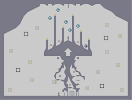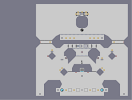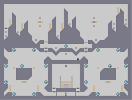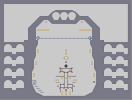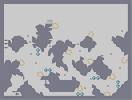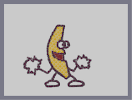A place where rocks fall up!!! Offset Symmetry Pleurisy Gladiator Dixlexic Carbon Miners Rule Rules!!!

Pages: (0)

### haha

this is soo cute =)

### heh

I actually dont like the shading.
and do you know why good n-artists get attention? because it's boring to do...

### Your

actually very good at n-arts, the shading is good

### Love the way you did the shading. ^^

The outer lines could have some more detail.
But overall it's cool. 4aved.
I'm glad I inspired some people to make arts ^^